# Python program to find the sum of n numbers

In this article, you will learn and get code to find the sum of "n" numbers entered by the user using a Python program. Here is the list of programs:

• Using a "for loop," find the sum of "n" numbers.
• Using a "while loop," find the sum of "n" numbers.
• Find the sum of "n" numbers using "list."
• Find the sum of "n" numbers using a user-defined "function."

For example, if the user enters the value of "n" as 3 and then three numbers as 1, 2, and 3, then the answer will be "1+2+3" or 6.

## Using a for loop, compute the sum of n numbers

This program finds the sum of "n" numbers using a "for loop." Here, the value of "n" and then "n" numbers must be entered by the user as shown in the program and its output given below:

```sum = 0
print("Enter the Value of n: ")
n = int(input())
print("Enter " + str(n) + " Numbers: ")
for i in range(n):
num = int(input())
sum = sum+num
print("Sum of " + str(n) + " Numbers = " + str(sum))```

Here is its sample run: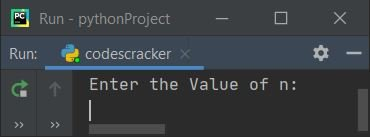Now supply the input, say 6 as the value of n, and then enter 6 numbers, say 10, 20, 30, 40, 50, and 60. Here is the sample output with exactly these inputs: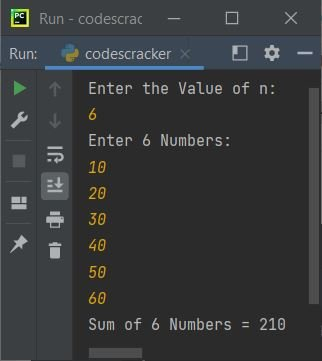Note: "str()" is used to convert from integer to string. Because the "+" operator in Python is used to concatenate only values of the same type. The following code

`for i in range(n):`

is used to execute the following block of code:

```num = int(input())
sum = sum+num```

n number of times, starting with the value of i from 0 to n-1. For example, if the user enters the value of n as 10, then this block of code gets executed 10 times. Using this block, we've received 10 numbers, which add their values and are initialized to "sum," one by one.

## Using a while loop, compute the sum of n numbers

This program does the same job as the previous program, using "while" loop instead of "for":

```sum = 0
i=0
print(end="Enter the Value of n: ")
n = int(input())
print(end="Enter " + str(n) + " Numbers: ")
while i<n:
num = int(input())
sum = sum+num
i = i+1
print("\nSum of " + str(n) + " Numbers = " + str(sum))```

Here is its sample run, with user input of 10 as the value of n and 1, 2, 3, 4, 5, 6, 7, 8, 9, and 10 as the 10 numbers: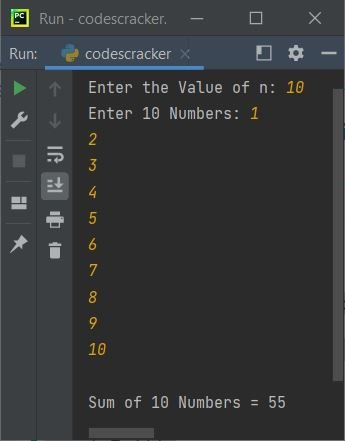## Sum of n numbers using a list (an array)

This program finds and prints the addition result (or sum) of "n" numbers entered by the user at run-time using "list." A list is like an array:

```num = []
sum = 0
print(end="Enter the Value of n: ")
n = int(input())

print(end="Enter " + str(n) + " Numbers: ")
for i in range(n):
num.insert(i, int(input()))

for i in range(n):
sum = sum+num[i]

print("\nSum of " + str(n) + " Numbers = " + str(sum))```

Here is its sample run with the user input, 5 as the value of n, and 5, 6, 7, 10, and 12 as five numbers: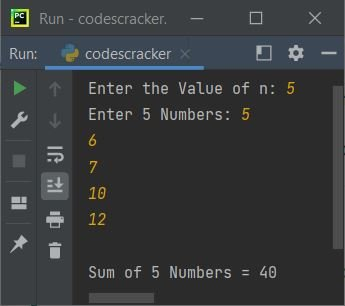The following code

`for i in range(n):`

is used to execute the following statement:

`num.insert(i, int(input()))`

n times with i values ranging from 0 to n-1. Therefore, using "insert()," the value entered by the user gets inserted into the list num[] one by one in this way:

• The first value gets stored in num[i] or num.
• The second value gets stored in num.
• The third value gets stored in num.
• and so on.

## Find the sum of n numbers using a function

This program is the last one in this article. This program also finds the sum of n numbers, as done in previous programs using different approaches. This program, like those, approaches the same task in a different way (a user-defined function):

```def SumOfNNums(arr, tot):
s = 0
for i in range(tot):
s = s+arr[i]
return s

num = []
print(end="Enter the Value of n: ")
n = int(input())
print(end="Enter " + str(n) + " Numbers: ")
for i in range(n):
num.insert(i, int(input()))

sum = SumOfNNums(num, n)
print("\nSum of " + str(n) + " Numbers = " + str(sum))```

Here is its sample run with user input, "3" as value of "n" and "20, 30, 10" as 3 numbers: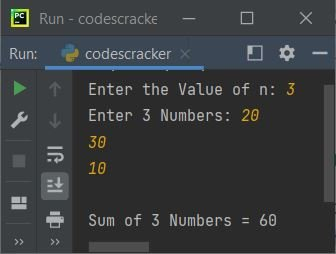with the following statement:

`sum = SumOfNNums(num, n)`

We've called the function "SumOfNNums()." So after executing this statement, the function gets executed, and the value returned by the function is the result, which is the sum of "n" numbers entered by the user. So the return value gets initialized to "sum," and its value gets printed.

Python Online Test

« Previous Program Next Program »